﻿ 岩溶大断面隧道开挖施工参数比选及现场实测分析 Simulation and Analysis of Construction Parameters in Karst Tunnel Excavation

Hans Journal of Civil Engineering
Vol. 08  No. 07 ( 2019 ), Article ID: 32362 , 8 pages
10.12677/HJCE.2019.87144

Simulation and Analysis of Construction Parameters in Karst Tunnel Excavation

Mingchao Yan

The Fourth Engineering Co. Ltd. of China Railway Five Council, Shaoguan Guangdong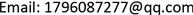Received: Sep. 4th, 2019; accepted: Sep. 20th, 2019; published: Sep. 27th, 2019ABSTRACT

The surrounding rock of a Karst tunnel of Huaishaoheng railway is soft and crushed, which uses three-step excavation method with temporary inverted arch and is easy to cause large deformation in surrounding rock and is not steady, so it’s very important to choose proper excavation construction parameters. Based on the Karst tunnel, it simulated deformation of surrounding rock and supported under different footage driving cycle and step length by using 3D elastic-plastic finite element method, compared the advantages and disadvantages of each excavation construction parameter, and provided a basis for proper excavation construction parameters selection. The results show that the excavation of Karst tunnel under V level surrounding rock can reduce the construction speed and increase the construction cost using 2 m excavation driving cycle and 6m step length, but it can control the deformation obviously and ensure the construction safety. Effectiveness is verified by the measured data obtained at site.

Keywords:Karst Tunnel, Excavation Construction Parameters, Driving Cycle, Step Length, Numerical Simulation, Deformation1. 引言

2. 工程概况

3. 数值模拟

3.1. 计算模型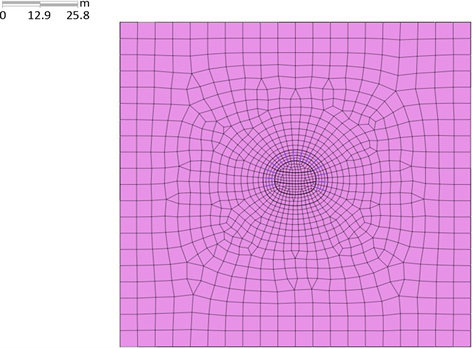Figure 1. Grid graph of the calculation model

3.2. 单元类型与屈服准则

① 单元类型的选取

② 塑性屈服准则

${\tau }_{n}=c-{\sigma }_{n}tg\phi$ (1)

${\sigma }_{n}$ ：剪切面上的法向应力，以拉为正；

$c,\phi$ ：岩土材料的粘聚力和内摩擦角。

$\frac{{\sigma }_{1}-{\sigma }_{3}}{2}=c\mathrm{cos}\phi -\frac{{\sigma }_{1}+{\sigma }_{3}}{2}\mathrm{sin}\phi$ (2)

$f\left({I}_{1},{J}_{2},\theta \right)=\frac{1}{3}{I}_{1}\mathrm{sin}\phi +\sqrt{{J}_{2}}\mathrm{sin}\left(\theta +\frac{\pi }{3}\right)+\frac{\sqrt{{J}_{2}}}{\sqrt{3}}\mathrm{cos}\left(\theta +\frac{\pi }{3}\right)\mathrm{sin}\phi -c\mathrm{cos}\phi =0$ (3)

3.3. 计算工况与参数Table 2. Physical and mechanics parameters of the calculation model

4. 不同施工参数数值模拟分析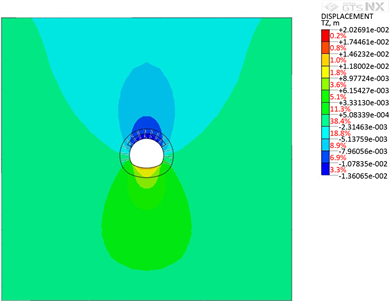Figure 2. Contour of vertical deformation of the surrounding rock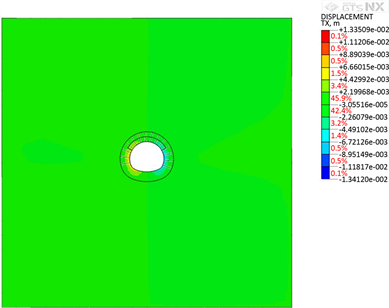Figure 3. Contour of horizontal deformation of the surrounding rock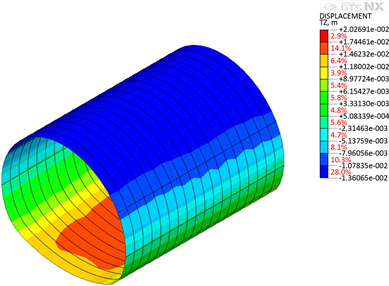Figure 4. Contour of vertical deformation of the tunnel lining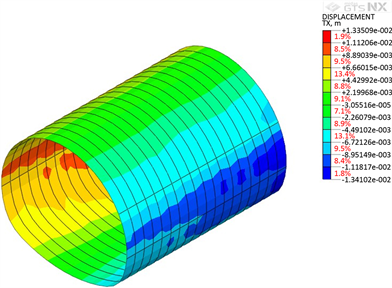Figure 5. Contour of horizontal deformation of the tunnel lining

5. 开挖支护方案比选分析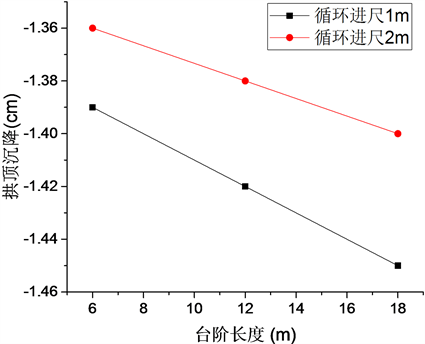Figure 6. Curve of vault settlement and step length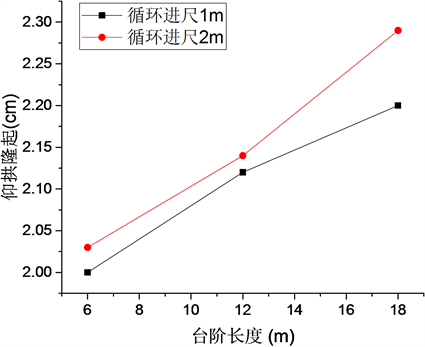Figure 7. Curve of inverted arch upfit and step length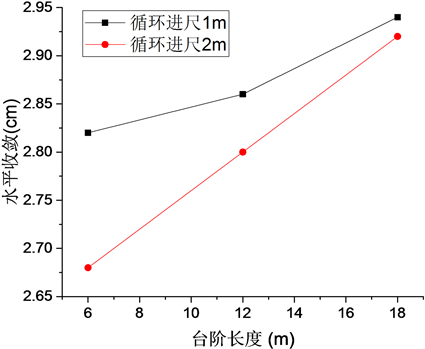Figure 8. Curve of level convergence and step lengthTable 3. Deformation of surrounding rock and support structure under different situation

6. 现场测试对比分析Table 4. Comparison of the site measured deformation and calculation value of surrounding rock

7. 结论

1) 对于岩溶隧道采用三台阶临时仰拱法开挖施工，循环进尺为2 m，台阶长度为6 m时，隧道拱顶沉降、仰拱隆起、水平收敛变形最大分别为−1.36 cm，2.03 cm，2.68 cm，在所有计算工况中围岩与衬砌变形最小。

2) 为避免第一步开挖当日变形量过大，在确定循环进尺时，需要考虑现场设备条件能否及时支护，为安全起见，可以选择1.5~2 m循环进尺；虽然隧道开挖台阶长度越小，其控制围岩变形能力越强，但短台阶空间局限性较大，施工工期较长。由于隧道围岩级别为V级，考虑施工过程安全以及施工工期的要求，最终选择隧道开挖循环进尺为2 m，台阶长度为6 m。

3) 现场测试结果显示：理论计算值与现场测试值基本吻合。工程实践证明：怀邵衡铁路岩溶隧道开挖施工时，采用三台阶临时仰拱法，选择循环进尺为2 m，台阶长度为6 m，可有效控制隧道受力和变形，确保岩溶隧道的施工安全。

Simulation and Analysis of Construction Parameters in Karst Tunnel Excavation[J]. 土木工程, 2019, 08(07): 1237-1244. https://doi.org/10.12677/HJCE.2019.87144

1. 1. 王晓. 断层破碎带地段隧道稳定性分析与施工参数优化研究[D]: [硕士学位论文]. 北京: 北京交通大学, 2017.

2. 2. 王君, 丁春林, 张亚龙, 王高彦, 舒进. 大断面浅埋隧道开挖施工技术及其数值模拟[J]. 施工技术, 2014, 43(S2): 120-123.

3. 3. 丁春林, 王君, 王高彦, 李志清. 浅埋大断面公路隧道开挖施工工法比选[J]. 现代隧道技术, 2014, 51(S1): 269-274.

4. 4. 仲崇海. 软弱围岩大断面隧道台阶法施工技术参数研究[D]: [硕士学位论文]. 北京: 北京交通大学, 2014.

5. 5. 黄大成. 软弱地层台阶七步开挖施工隧道围岩稳定与变形特征研究[D]: [硕士学位论文]. 长沙: 中南大学, 2010.

6. 6. 李贻伟. 岩溶公路隧道围岩–支护结构受力特性数值模拟分析[D]: [硕士学位论文]. 重庆: 重庆交通大学, 2010.

7. 7. 彭川. 岩溶隧道围岩与结构稳定性分析[D]: [硕士学位论文]. 长沙: 长沙理工大学, 2009.

8. 8. 李奎. 隧道岩溶围岩力学特性的初步研究[D]: [硕士学位论文]. 成都: 西南交通大学, 2005.